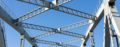# Yield Strength – All You need to know

Yield strength Re is a material parameter. It indicates the maximum mechanical stress up to which a material can be deformed under an uniaxial load without undergoing permanent plastic deformation. The material characteristic value is determined in a tensile test, but cannot be specified precisely in this way for many materials, such as low-alloy steels. Therefore, the 0.2% proof stress is used instead.

If the yield strength is exceeded, visible and permanent deformation occurs and components and materials can no longer be used safely. This is defined as the elastic limit.

## How is the yield strength determined for steels?

Due to Cottrell clouds, the yield strength of some materials cannot be determined exactly. This results in the formation of a pronounced yield strength and the distinction between lower and upper yield strength. This phenomenon occurs only in low-alloy and unalloyed steel grades.### Upper yield strength

The upper yield strength ReH> refers to the load at which plastic deformation of a material begins. It is also the highest stress before an initial drop and yielding of the stress begins. The lowest point here is the lower yield point.

The upper yield point can be determined using the stress-strain diagram

### Lower yield point

The lowest stress during yielding after reaching the upper yield point is called the lower yield point ReL. It is a result of dislocations breaking loose in the Cottrell clouds. Transient stresses must not be taken into account in the calculation.

### Lüders strain

Lüders strain is a yield strength effect that occurs only when the yield strength is pronounced. Lüders strain is the plastic strain component that occurs due to the dislocation movement through the material at a constant load. In the stress-strain diagram, the Lüders strain can be recognized by its zigzag shape. The emerging dislocations leave visible traces on the surface of the material, which are called Lüders bands.

## What is a proof strength?

The proof strength is also known as the equivalent yield point and can be precisely determined with the aid of the stress-strain diagram. It is therefore used for materials that do not have a pronounced yield point. Since in such materials there is a constant change between an elastic and plastic range, the 0.2% proof strength is used. This is the stress at which, after unloading, a permanent elongation of 0.2% is present.

The 0.2% proof strength is used for cold-rolled and cold-formed materials because they do not have a pronounced yield strength. In the case of highly ductile materials, such as plastics, a 2% proof strength is sometimes specified because the viscoplasticity of such materials means that the 0.2% yield strength can only be determined inadequately.

## Yield strength ratio

The yield strength ratio indicates how much a material can be elastically stressed and is the quotient of yield strength and tensile strength. The ratio is a measure of the deformability of a material. Materials with a high deformation capacity show a low yield strength ratio.